# Argand Plane and Polar Representation

#### description

• Representation of Complex Number - Argand Plane Representation
• Representation of Complex Number - Polar Representation of Complex Numbers

#### notes

Some complex numbers such as 2 + 4i, – 2 + 3i, 0 + 1i, 2 + 0i, – 5 –2i and 1 – 2i which correspond to the ordered pairs (2, 4), ( – 2, 3), (0, 1), (2, 0), ( –5, –2), and (1, – 2), respectively, have been represented geometrically by the points A, B, C, D, E, and F, respectively in the Fig.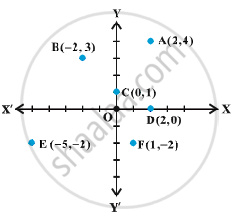The plane having a complex number assigned to each of its point is called the complex plane or the Argand plane.
x + iy= sqrt (x^2+ y^2)  is the distance between the point P(x, y) and the origin O (0, 0)
(Fig)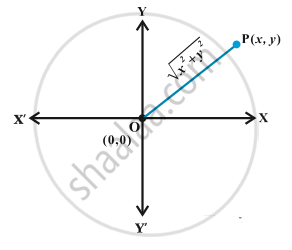The x-axis and y-axis in the Argand plane are called, respectively, the real axis and the imaginary axis.
The representation of a  complex number z = x + iy and its conjugate z = x – iy in the Argand plane are, respectively, the points P (x, y) and Q (x, – y).
Geometrically, the point (x, – y) is the mirror image of the point (x, y) on the real axis (Fig)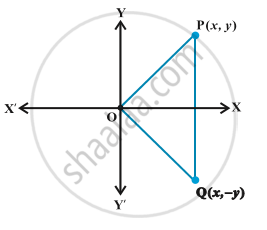Polar representation of a complex number:
Let the point P represent the nonzero complex number z = x + iy. Let the directed line segment OP be of length r and θ be the angle which OP makes with the positive direction of x-axis (Fig)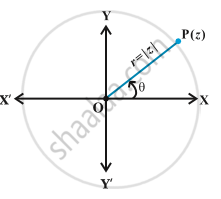We may note that the point P is uniquely determined by the ordered pair of real numbers (r, θ), called the polar coordinates of the point P.
We have, x = r cos θ, y = r sin θ and therefore, z = r (cos θ + i sin θ). The latter is said to be the polar form of the complex number. Here r= sqrt (x^2+ y^2)= |z|  is the modulus of z and θ is called the argument (or amplitude) of z which is denoted by arg z.
We shall take the value of θ such that – π <  θ ≤  π, called principal argument of z and is denoted by arg z, unless specified otherwise.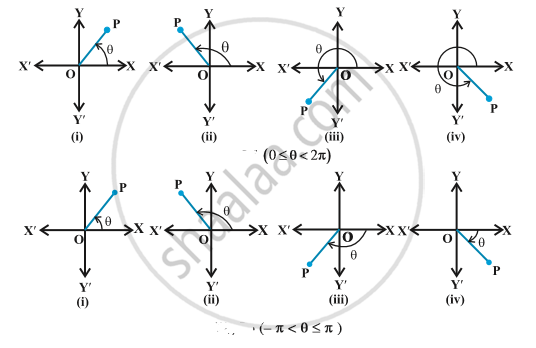If you would like to contribute notes or other learning material, please submit them using the button below.

### Shaalaa.com

Argand and polar form of complex number [00:11:00]
S
0%